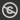Science, Maths & Technology
Free course

# Bayesian statistics## Course reviews

This free course is an introduction to Bayesian statistics. Section 1 discusses several ways of estimating probabilities. Section 2 reviews ideas of conditional probabilities and introduces Bayes’ theorem and its use in updating beliefs about a proposition, when data are observed, or information becomes available. Section 3 introduces the main ideas of the Bayesian inference process. The prior distribution summarises beliefs about the value of a parameter before data are observed. The likelihood function summarises information about a parameter contained in observed data and the posterior distribution represents what is known about a parameter after the data have been observed.

## Course learning outcomes

After studying this course, you should be able to:

• use relative frequencies to estimate probabilities
• calculate conditional probabilities
• calculate posterior probabilities using Bayes' theorem
• calculate simple likelihood functions
• describe the role of the posterior distribution, the likelihood function and the posterior distribution in Bayesian inference about a parameter Ɵ.

First Published: 19/12/2017

Updated: 22/10/2018

Skip Rate and Review